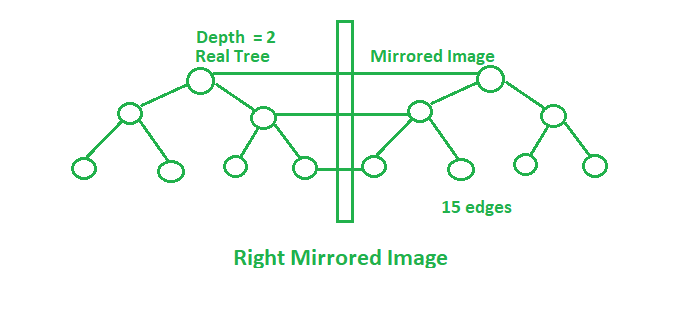# Number of edges in mirror image of Complete binary tree

• Difficulty Level : Basic
• Last Updated : 28 May, 2021

Given a complete binary tree of depth H. If the mirror image from the left and the right side of this tree is taken then:

Right Mirrored Image: Rightmost node of the every level is connected to mirrored corresponding node.
Left Mirrored Image: Left most node of the every level is connected to mirrored corresponding node.

The task is to find the number of edges after taking both the mirror images in the final tree.Examples:

Input: H = 1
Output: 10
2 edges in the original tree will get mirrored in the mirror images (left and right) i.e. 6 edges in total.
And the edges connecting the mirror images with the original tree as shown in the image above.
Input: H = 2
Output: 24
(6 * 3) + 3 + 3 = 24

Approach: Maintain the leftmost, rightmost nodes after each mirror image. Number of edges will change after each operation of mirror image. Initially,After right mirrored image:After left mirrored image:In complete modified tree:Below is the implementation of the above approach:

## C++

 // C++ implementation of the approach #include  using namespace std;   // Function to return the total number // of edges in the modified tree int countEdges(int H) {       int edges, right, left;     edges = 2 * (pow(2, H) - 1);     left = right = H + 1;       // Total edges in the modified tree     int cnt = (edges * 3) + left + right;     return cnt; }   // Driver code int main() {     int H = 1;       cout << countEdges(H);       return 0; }

## Java

 // Java implementation of the approach import java.io.*;   class GFG {       // Function to return the total number     // of edges in the modified tree     static int countEdges(int H)     {           int edges, right, left;         edges = 2 * (int)(Math.pow(2, H) - 1);         left = right = H + 1;           // Total edges in the modified tree         int cnt = (edges * 3) + left + right;         return cnt;     }       // Driver code     public static void main(String[] args)     {         int H = 1;         System.out.println(countEdges(H));     } }   // This code has been contributed by anuj_67..

## Python 3

 # Python 3 implementation of the approach   # Function to return the total number # of edges in the modified tree def countEdges( H):       edges = 2 * (pow(2, H) - 1)     left = right = H + 1       # Total edges in the modified tree     cnt = (edges * 3) + left + right     return cnt   # Driver code if __name__ == "__main__":     H = 1;       print(countEdges(H))   # This code is contributed by ChitraNayal

## C#

 // C# implementation of the approach  using System;   class GFG  {        // Function to return the total number      // of edges in the modified tree      static int countEdges(int H)      {            int edges, right, left;                    edges = 2 * (int)(Math.Pow(2, H) - 1);          left = right = H + 1;            // Total edges in the modified tree          int cnt = (edges * 3) + left + right;          return cnt;      }        // Driver code      public static void Main()      {          int H = 1;          Console.WriteLine(countEdges(H));      }    }    // This code is contributed by AnkitRai01

## Javascript

 

Output:

10

My Personal Notes arrow_drop_up
Recommended Articles
Page :